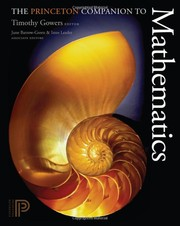Le pouvoir des mathématiques - ISSN ? - Les Dossiers De La Recherche November 2009

Motivation

Describe in a sentences or two what motivated me to read this book.

Draw a schema (using PmGraphViz or another solution) of the situation of the area in the studied domain before having read the book.

• 1 Introduction to Template
• "interesting quote." (p1)
• note
• ...
• 2 Interesting thing
• (p20)

L'arbre de la complexite

Dossier : "3 L'arbre de la complexité", La Recherche 2010

• Probleme ouvert
• de Hilbert a Clay
• Non formule
• les equations de la mecanique des fluides
• Conjecture
• De nature inconnue
• l'hypothese de Riemann
• Indecidable
• le 2eme probleme de Hilbert
• Decidable
• Preuve avec un contre-exemple
• le 3eme probleme de Hilbert
• Theoreme
• Autres theoremes
• le grand theoreme de Fermat
• l'hypothese de Poincarre
• Theoreme d'existence
• Demonstration par l'absurde
• e n'est pas un rationel
• Constructif
• Methode
• Complexite inconnue
• le voyageur de commerce
• Complexite non polynomiale
• l'arithmetique de Presburger
• Complexite polynomiale
• Grand degre
• Ce nombre est-il premier ?
• Petit degre
• Le plus grand commun diviseur

See also Complexity class in Wikipedia and Bounded error, Quantum, Polynomial time(for P=QP) in the new concept page.

Overall remarks and questions

• this? that?

Synthesis

So in the end, it was about X and was based on Y.

Critics

Point A, B and C are debatable because of e, f and j.

Vocabulary

(:new_vocabulary_start:) new_word (:new_vocabulary_end:)